Go back to  'Maths'

We at Cuemath believe that Math is a life skill. Our Math Experts focus on the “Why” behind the “What.” Students can explore from a huge range of interactive worksheets, visuals, simulations, practice tests, and more to understand a concept in depth.

Book a FREE trial class today! and experience Cuemath’s LIVE Online Class with your child.

## Introduction

The word "Quadratic" is derived from the word "Quad" which means square.

In other words, a quadratic equation is an “equation of degree 2.”

There are many scenarios where quadratic equations are used.

Did you know that when a rocket is launched, its path is described by a quadratic equation?## Definitions and Examples

An equation of the form $$ax^2+bx+c=0$$, where $$a \neq 0$$ is called a quadratic equation.

Here are some examples of equations:

Equations Values of $$a$$, $$b$$ and $$c$$
1. $$8x^2+7x-1=0$$ \begin{align}a&=8 \\b&=7 \\c&=-1 \end{align}
2. $$4x^2-9=0$$ \begin{align}a&=4 \\b&=0 \\c&=-9 \end{align}
3. $$x^2+x=0$$ \begin{align}a&=1 \\b&=1 \\c&=0 \end{align}
4. $$6x-8=0$$ \begin{align}a&=0 \\b&=6 \\c&=-8 \end{align}

Look at Example 4.

Here, $$a = 0$$. Therefore, it is not a quadratic equation.

The remaining equations are quadratic equation examples.

## Standard Form and Hidden Quadratic Equations

You are now aware of the standard form of a quadratic equation.

It looks like this: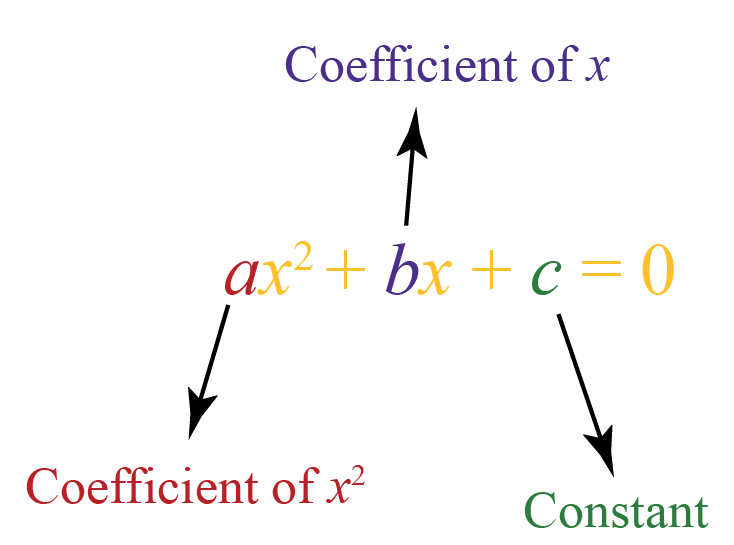This is a quadratic equation in variable $$x$$.

But always remember that $$a$$ is a non-zero value.

Sometimes, a quadratic equation is NOT written in its standard form.

Here are some quadratic equation examples:

 Equations Steps to follow Standard Form $$(x-1)(x+2)=0$$ Multiply the factors $$(x-1)$$ and $$(x+2)$$ $$x^2+x-2=0$$ $$-x^2=-3x+1$$ Move the term $$-x^2$$ to the right side of the $$=$$ sign $$x^2-3x+1=0$$ $$5x(x+3)=12x$$ Expand the bracket on the left side and move $$12x$$ to the left side of the $$=$$ sign $$5x^2+3x=0$$ $$x^3=x(x^2+x-3)$$ Expand the bracket on the right side and move $$x^3$$ to right side of the $$=$$ sign $$x^2-3x=0$$

Have a doubt that you want to clear? Get it clarified with simple solutions on Quadratic Equations from our Math Experts at Cuemath’s LIVE, Personalised and Interactive Online Classes.

Make your kid a Math Expert, Book a FREE trial class today!

### How to solve Quadratic Equations?

Let’s sharpen our skills by solving some quadratic equation problems.

We’ll do a bunch of examples on solving quadratic equations by factorisation.

Example 1:

$4x-12x^2=0$

Given any quadratic equation, first check for common factors.

In this example, check for the common factors among $$4x$$ and $$12x^2$$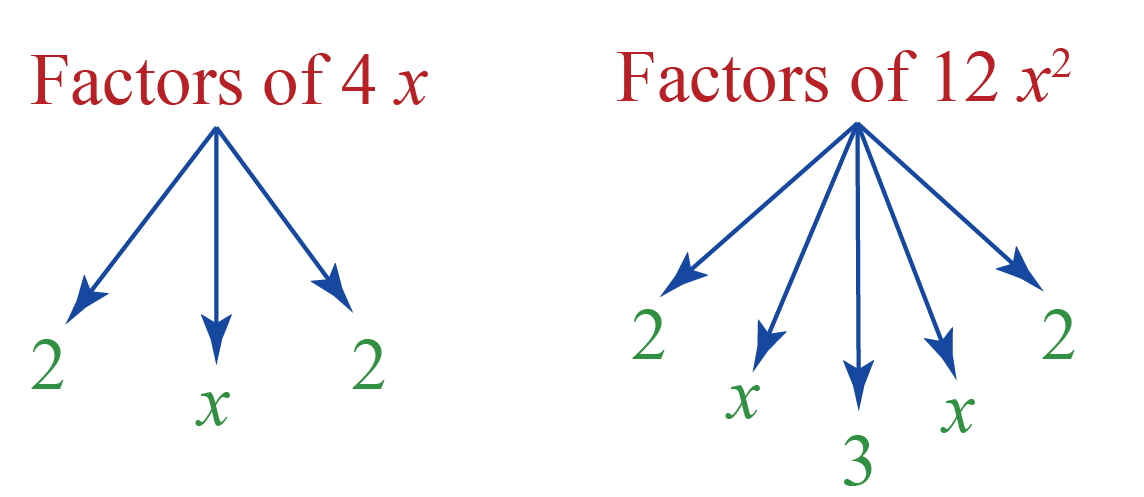We can observe that $$4x$$ is a common factor. Let’s take that common factor from the quadratic equation.

\begin{align}4x-12x^2&=0\\4x(1-3x)&=0\end{align}

Thus, by factoring the quadratic equation, we get the equation

$4x-12x^2=0$

Example 2:

$x^2+5x+6=0$

We’ll factor this in two binomials, namely $$(x+a)$$ and $$(x+b)$$.

Expand $$(x+a)(x+b)=0$$.

\begin{align}(x+a)(x+b)&=0\\x(x+b)+a(x+b)&=0\\x^2+bx+ax+ab&=0\\x^2+(a+b)x+ab&=0\end{align}

Let’s try to find $$a$$ and $$b$$ such that $$(a+b)$$ is mapped to 5 and $$ab$$ is mapped to 6.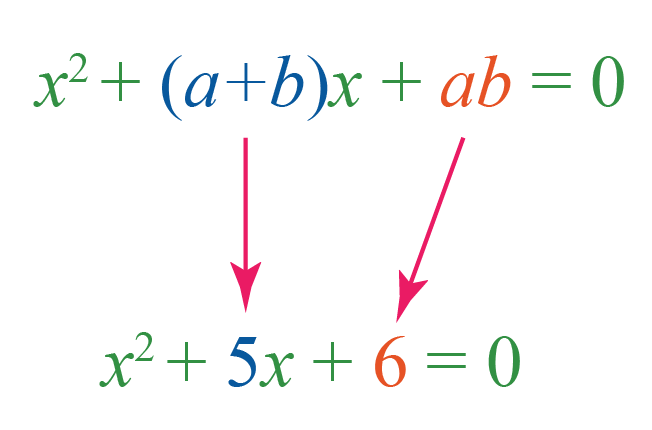The factors of $$6$$ are $$1$$, $$2$$, $$3$$ and $$6$$. Find the pairs $$a$$ and $$b$$ from $$1$$, $$2$$, $$3$$ and $$6$$ such that $$a+b=5$$ and $$ab=6$$

This is the right pair: $$2$$ and $$3$$, because, $$2+3 = 5$$ and $$2 \times 3=6$$

Thus, the quadratic equation can be factored as

$(x+2)(x+3)=0$

Example 3:

$2x^2+3x+1=0$

Dealing with the quadratic equation example $$2x^2+3x+1=0$$ is different because the product $$(x+a)(x+b)$$ gives 1 as the coefficient of $$x^2$$

In this example, you’ll use the general way to factorise the quadratic equation.

Find the values of $$a$$ and $$b$$ such that their product is the product of coefficient of $$x^2$$ and the constant and their sum is the coefficient of $$x$$.

Mathematically, $$ab=2$$ and $$a+b=3$$.

It’s not difficult to say that $$a=2$$ and $$b=1$$.

The quadratic equation can be reduced in the way,

\begin{align}2x^2+3x+1&=0\\2x^2+2x+x+1&=0\\2x(x+1)+(x+1)&=0\x+1)(2x+1)&=0\end{align} \(2x^2+3x+1=0 can be written as

$(x+1)(2x+1)=0$

## About Formula and Rhyme to Remember the Formula

Sometimes working with a hard quadratic equation is difficult. But don’t worry! Quadranator is here to help.

Quadranator alone is enough to hunt down all the solutions of the quadratic equations.

Here it is: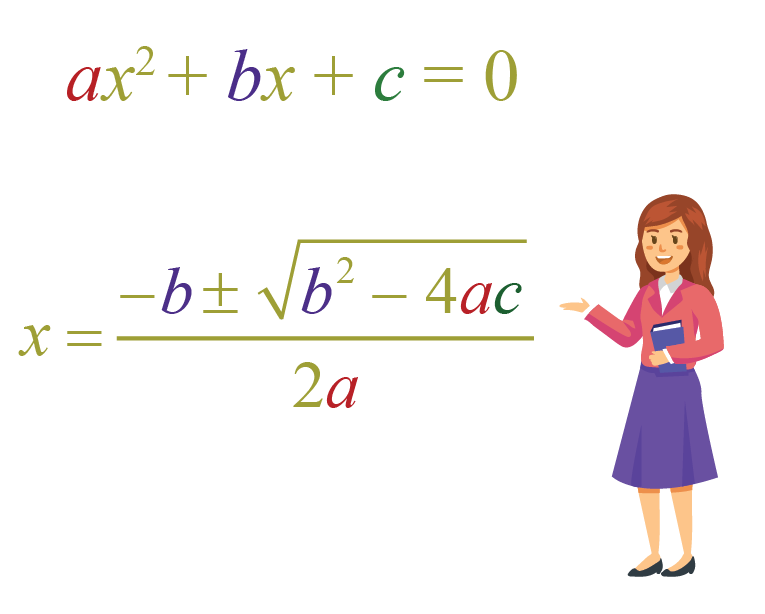This is also called a quadratic formula.

### Sing Together to Remember the Formula

Now, let’s memorize the quadratic equation formula. A rhyme or a song is a quick way to do that.

So, just sing along with the tune of “Pop Goes the Weasel.”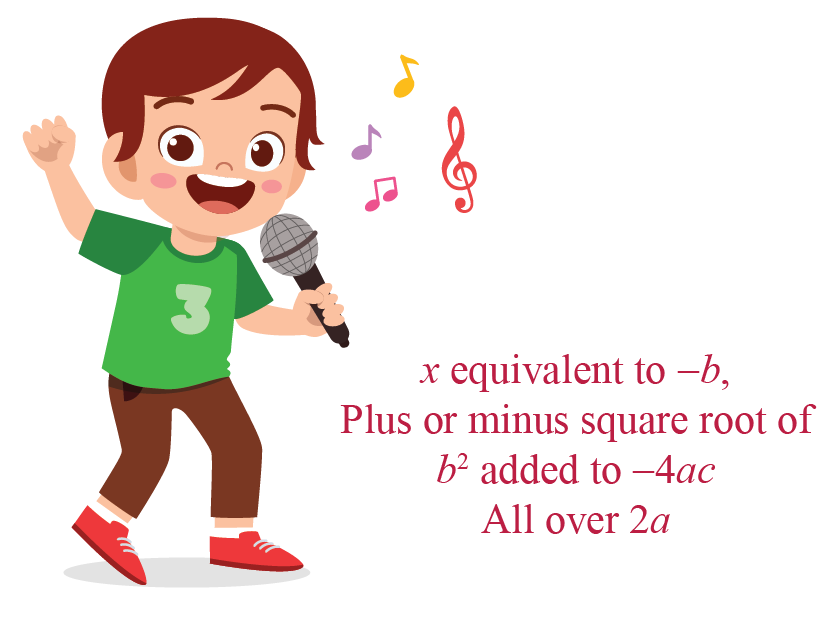A quadratic equation has at most two solutions.

Plug in the values of $$a$$, $$b$$ and $$c$$ in the quadratic equation formula to find the two solutions.

But, does every quadratic equation have two different solutions?

It might be possible that both solutions are the same or the solution doesn’t even exist.

It’s not really difficult to determine the nature of solutions.

### Discriminant

Do you observe the term $$b^2-4ac$$ in quadranator?

This is called a "discriminant" because it discriminates the quadratic equation solutions based on its sign.

• $$b^2-4ac>0$$

In this case, the quadratic equation has two distinct real roots.

• $$b^2-4ac=0$$

In this case, the quadratic equation has one repeated real root.

• $$b^2-4ac<0$$

In this case, the quadratic equation has no real root.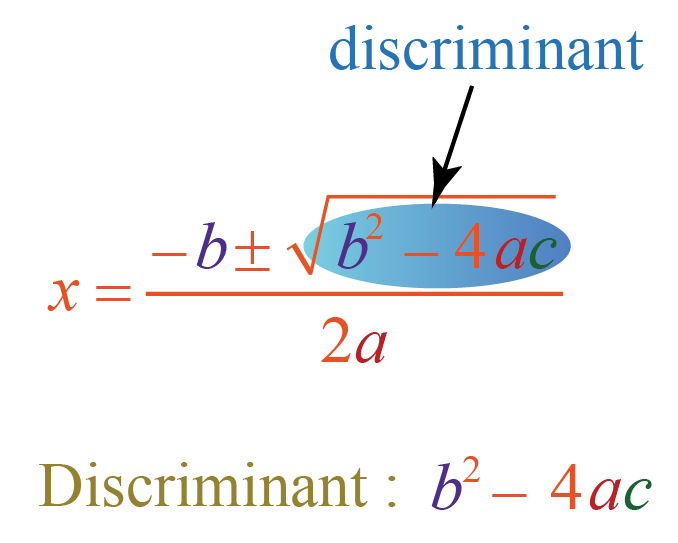This is how the quadratic equation is represented on a graph.

This curve is called a parabola.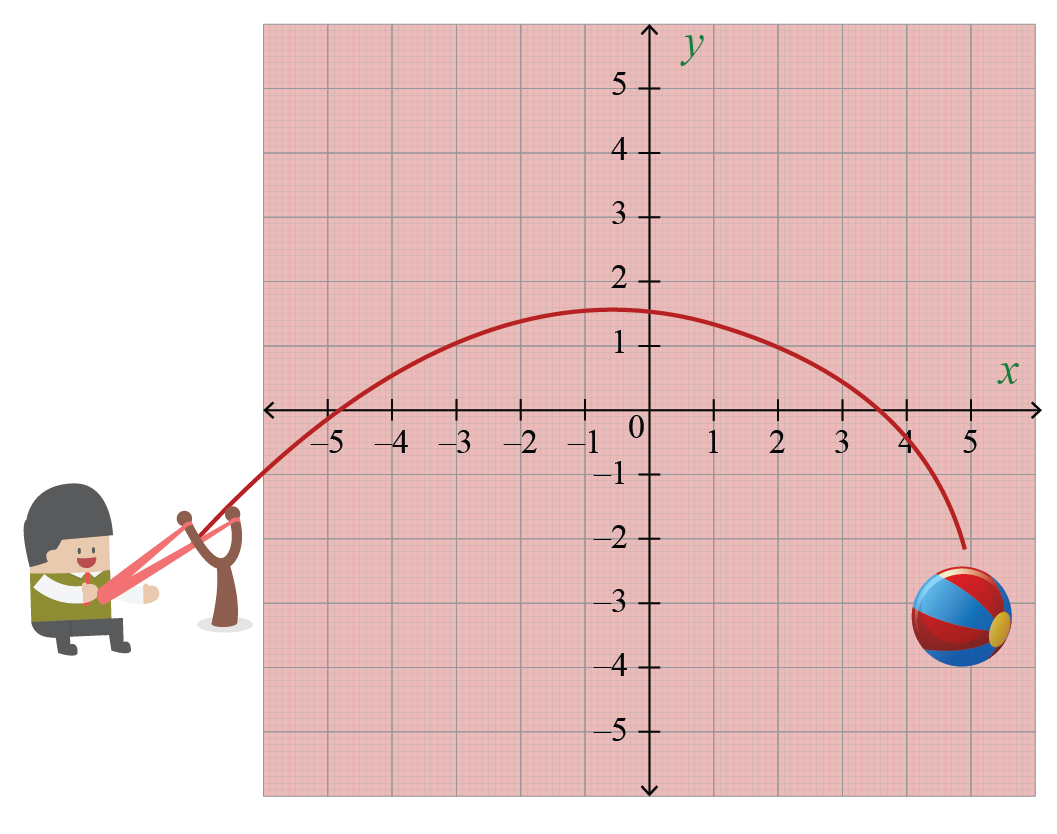Now let’s explore some quadratic equations on graph using the simulation below.

Drag the values of $$a$$ to check for the variations of upward parabola and downward parabola.

Dragging the values of $$b$$ will move the curve either right or left.

Dragging the values of $$c$$ will move the curve either up or down.

The roots of a quadratic equation are the same as its solutions.

This means that the quadratic equation roots are the values of $$x$$ for which the quadratic equation equals zero.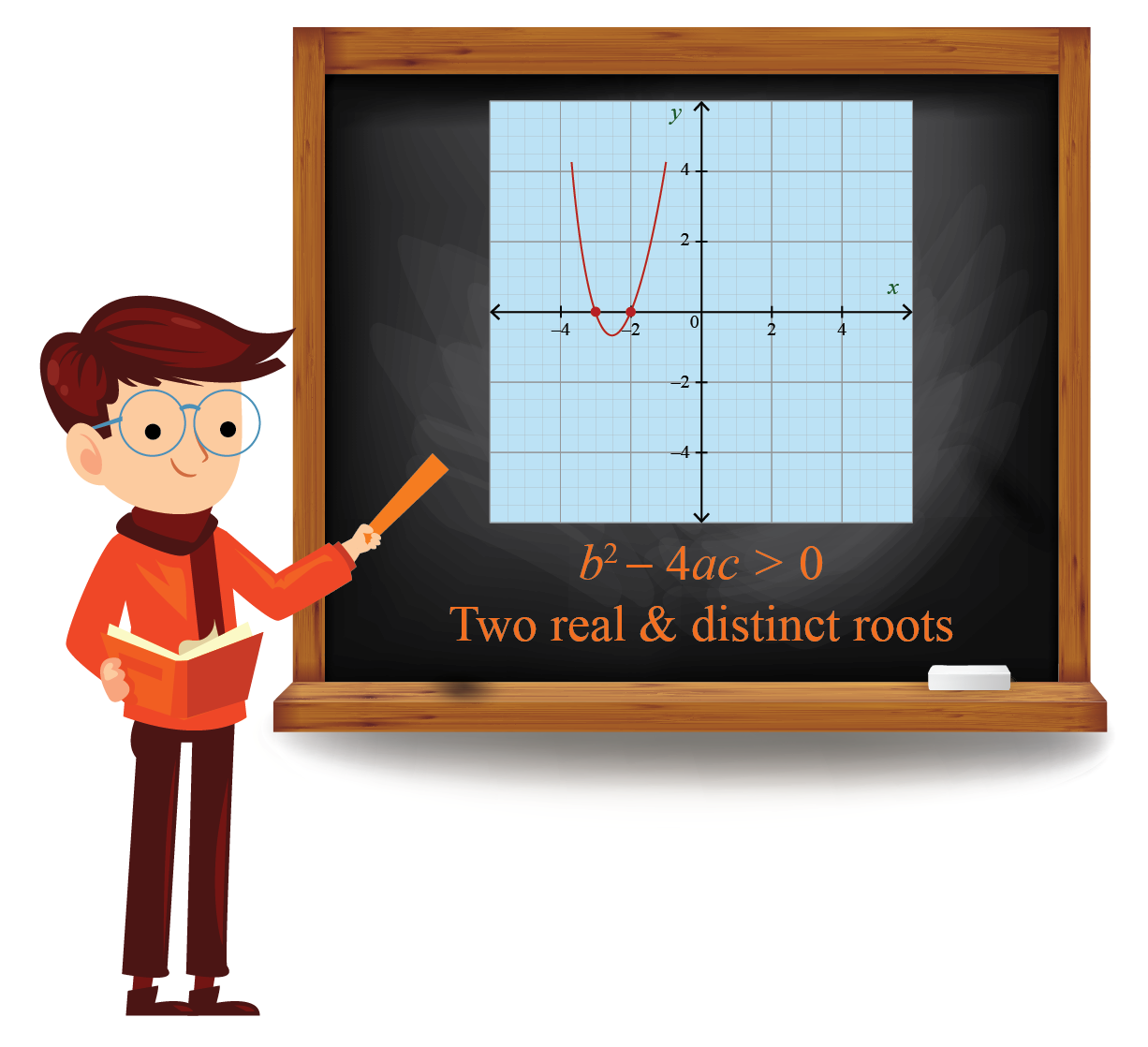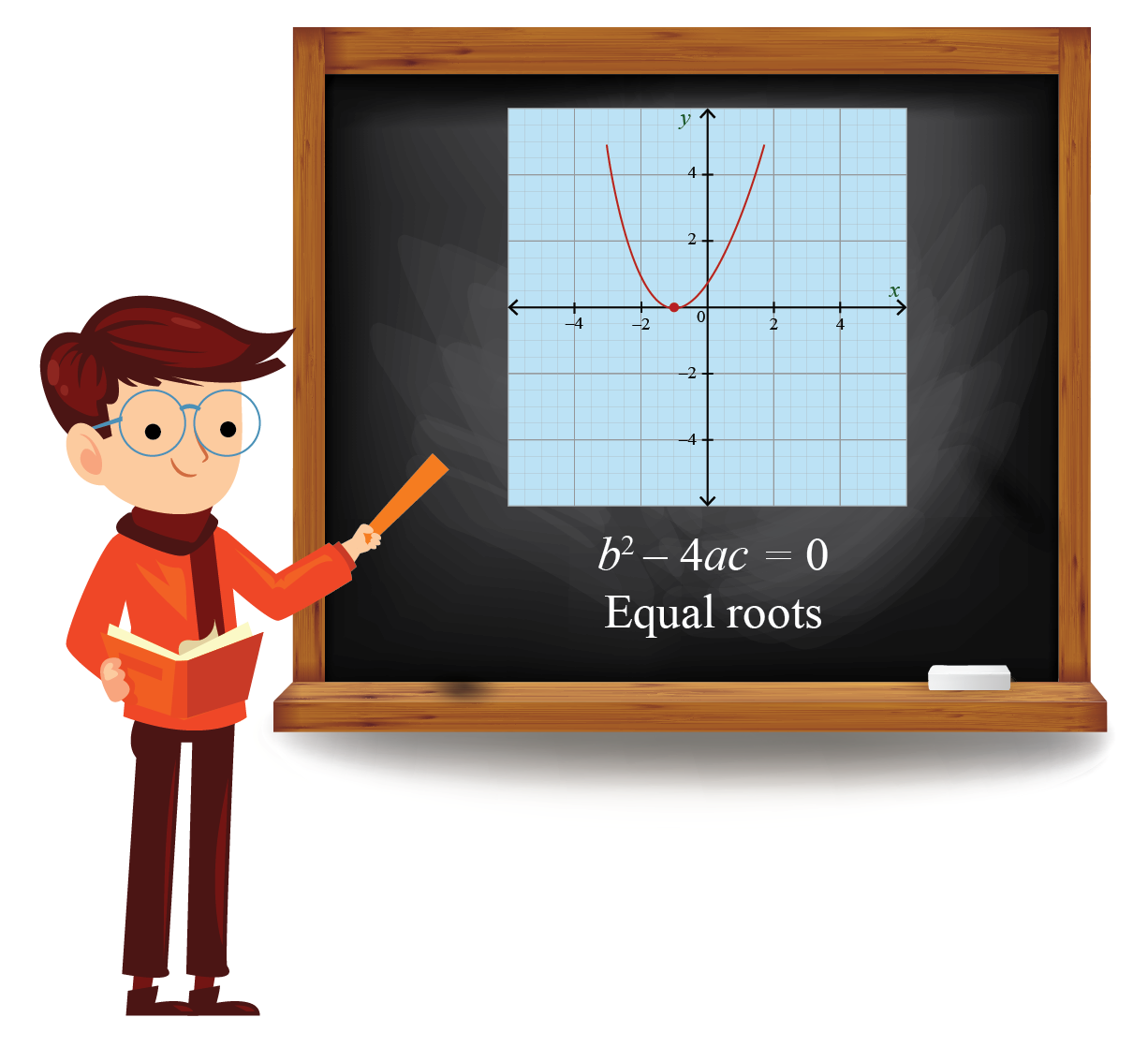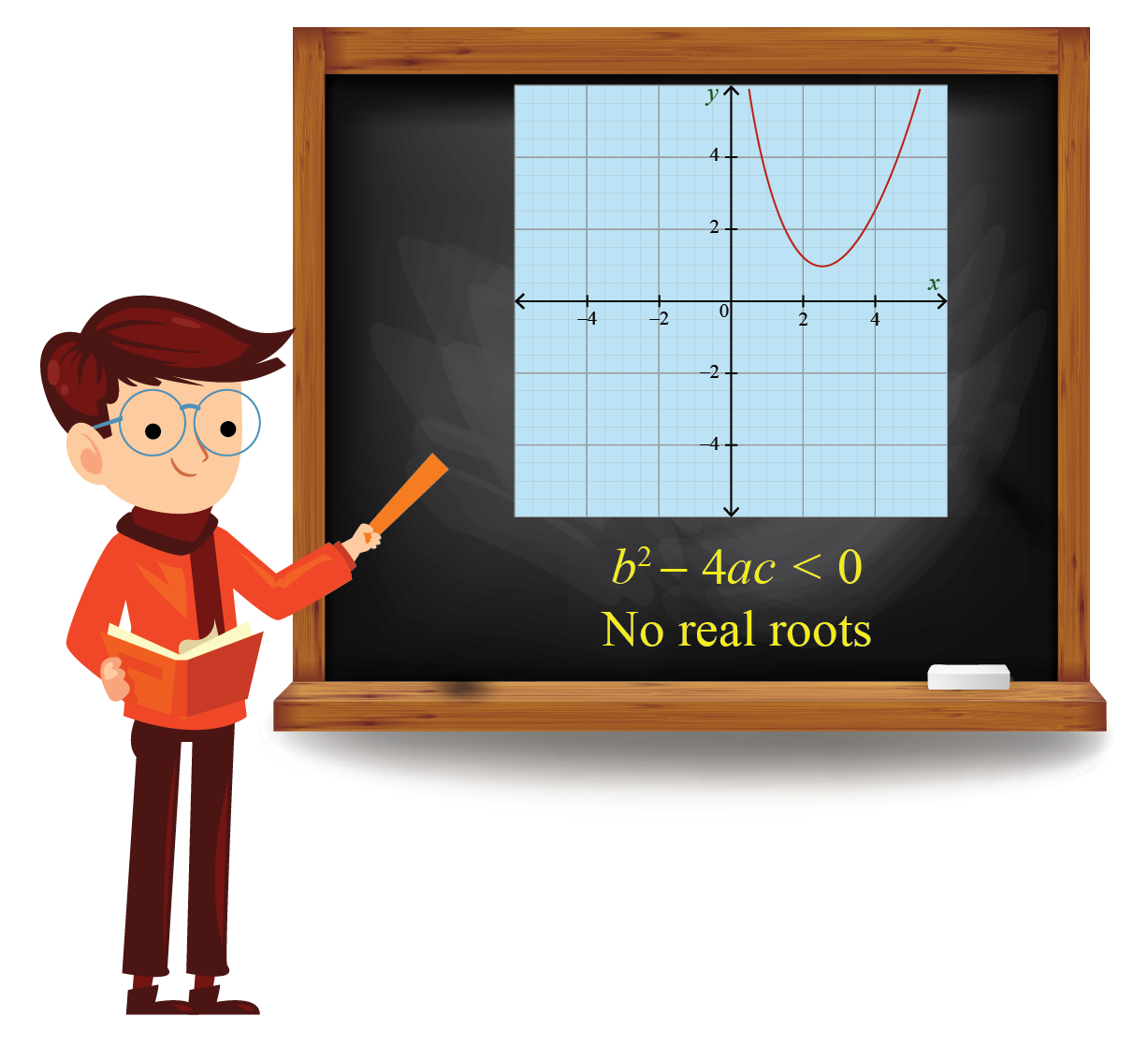### Sum of Roots of Quadratic Equations

If $$\alpha$$ and $$\beta$$ are the two roots of a quadratic equation, $$x^2+bx+c=0$$, the sum of roots is equal to negative of $$b$$ and the product of roots is equal to the constant term $$c$$.

Mathematically, this forms the following two equations:

 $$\alpha + \beta = -b$$

and

 $$\alpha \cdot \beta =c$$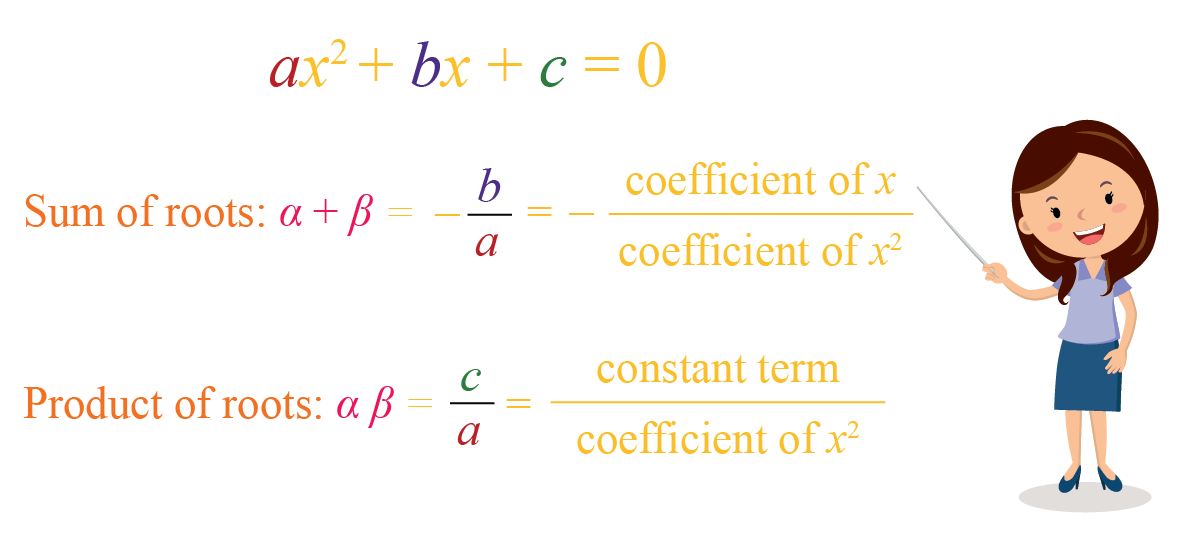Let us try and solve a few quadratic equations of class 10.

## Methods to Solve a Quadratic Equation

Let us consider the quadratic equation

$2x^2+x-3=0$

You can observe that this equation has no common factor.

Let’s find the determinant to find the nature of the solution.

\begin{align}b^2-4ac&=1^2-4\times 2\times (-3)\\&=1+24\\&=25>0\end{align}

This means that the quadratic equation has two real and distinct roots.Let's solve $$2x^2+x-3=0$$ quadratic equation with 2 different methods:

1. by Factorisation
2. by Formula

### Method 1: By Factorisation

Let’s solve this quadratic equation by factorisation method.

Here, we need to find $$a$$ and $$b$$ such that $$a+b=1$$ and $$ab=-6$$

Considering this, we see that the numbers are $$a=3$$ and $$b=-2$$
\begin{align}2x^2+x-3&=0\\2x^2+3x-2x-3&=0\end{align}

Take the common factors out from the first two terms and last two terms.

So, this quadratic equation factoring gives:
\begin{align}x(2x+3)-(2x+3)&=0\2x+3)(x-1)&=0\end{align} When the product of two numbers is \(0, then either of the numbers are $$0$$

This means $$2x+3=0$$ or $$x-1=0$$

Solving these two equations gives

 \begin{align} x=-\frac{3}{2},1 \end{align}

### Method 2: By Formula

Now, let’s use the quadratic formula to solve this quadratic equation of class 10.

Just plug in the values $$a=2$$, $$b=1$$ and $$c=-3$$ in the quadratic formula.

\begin{align}x&=\frac{-b\pm \sqrt{b^2-4ac}}{2a}\\&=\frac{-1\pm \sqrt{1^2-4\times 2\times (-3)}}{2\times2}\\&=\frac{-1\pm \sqrt{25}}{4}\\&=\frac{-1\pm 5}{4}\\&=-\frac{3}{2},1\end{align}

 \begin{align} x=-\frac{3}{2},1 \end{align}Think Tank
1. Is it possible to construct a rectangular park with perimeter $$60\;\text{m}$$ and area $$200\;\text{sq. m}$$. If yes, what are its length and breadth?
2. The sum of two numbers is 45. After subtracting 5 from both numbers, the product of the numbers is 124. What are the numbers?

Help your child score higher with Cuemath’s proprietary FREE Diagnostic Test. Get access to detailed reports, customized learning plans, and a FREE counseling session. Attempt the test now

## Solved Examples

There are a number of quadratic equation questions that will show you the real-world situations dealing with quadratic equations.

 Example 1

Mitali is a fitness enthusiast and goes for a jog every morning.

The park where she jogs is rectangular in shape and measures $$12\;\text{m}$$ by $$8\;\text{m}$$.

An environmentalist group plans to revamp the park and decides to build a pathway surrounding the park.

This would increase the total area to $$140\;\text{sq. m.}$$ What will be the width of the pathway?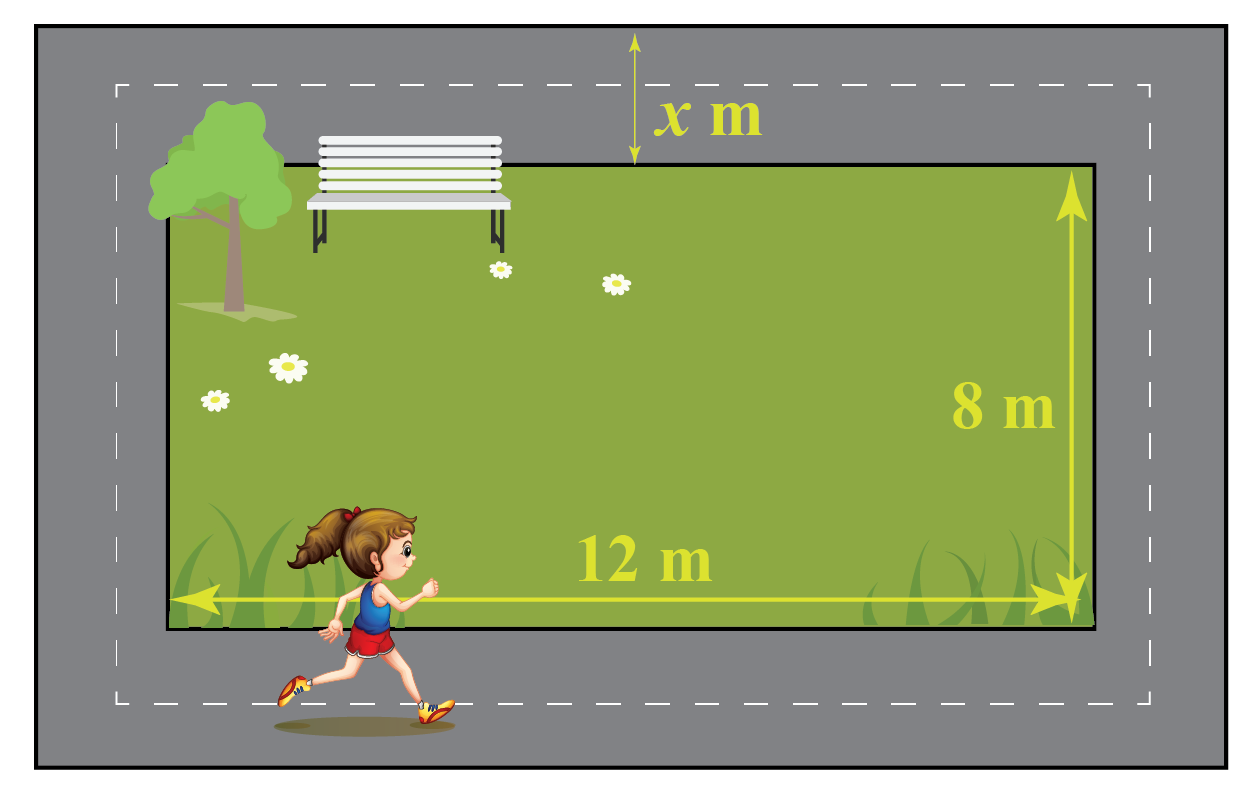Solution:

Let’s denote the width of the pathway by $$x$$.

Then, the length and breadth of the outer rectangle is $$(12+2x)\;\text{m}$$ and $$(8+2x)\;\text{m}$$.

According to the question,
\begin{align}(12+2x)(8+2x)&=140\\2(6+x)\cdot 2(4+x)&=140\x+6)(x+4)&=35\\x^2+10x-11&=0\\x^2+11x-x-11&=0\\x(x+11)-(x+11)&=0\\(x+11)(x-1)&=0\\x&=1,-11\end{align} Since length can’t be negative, we take \(x=1

 $$\therefore$$ Width of the pathway  $$= 1\;\text{m}$$
 Example 2

The next quadratic equation question will show you its application in the area of motion.

Hema throws a ball upwards from a platform which is  $$20\;\text{m}$$ above the ground.

The height of the ball from the ground at time '$$t$$', is denoted by '$$h$$'. Suppose

$h = -4t^2+16t+20$

Find the maximum height attained by the ball.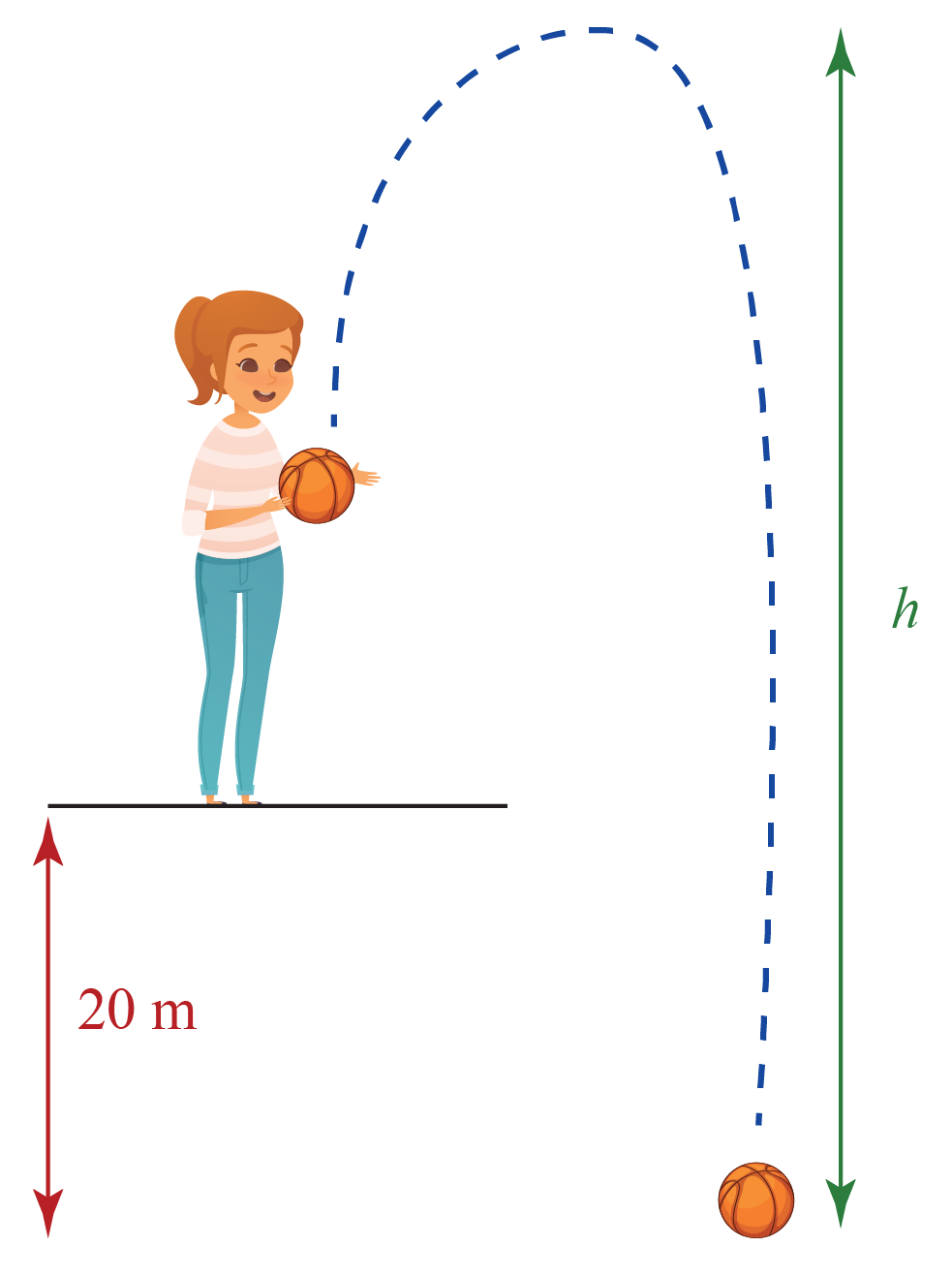Solution:

We can rearrange the terms of the quadratic equation

$h = -4t^2+16t+20$

in such a way that it is easy to find the maximum value of this equation.

\begin{align}h &= -4t^2+16t+20\\&= -4t^2+16t+20\\&=-4(t^2-4t-5)\\&=-4((t-2)^2-9)\\&=-4(t-2)^2+144\end{align}

We should keep the value of $$(t-2)^2$$ minimum in order to find the maximum value of $$h$$.

So, the minimum value $$(t-2)^2$$ can take is 0.

 $$\therefore$$ Maximum height attained $$= 144\;\text{m}$$

## Summary

Now, let's summarise the entire lesson. Below are the revision notes for you.

### Practice Problems on Quadratic Equations

1. The sum and product of zeros of a quadratic polynomial f(x) are S = -8/3 and P = 4/3 respectively. Write the correct expression for f(x) if its constant term is 4.

1. 3x2 + 8x + 4
2. 3x2 + 8x - 4
3. 3x2-8x + 4
4. 8x2 + 3x + 4

2. If (x + 4) is the factor of the equation px2 + 6x + 8q = 0, then which of the following relations is correct?

1. p - q = 3
2. 2p - q = 4
3. 2p + q = 3
4. p + 2q = 6

3. The sum of the squares of the zeros of x2 - 8x + p = 0 is 40. What is the value of p?

1. 12
2. 8
3. 120
4. 3

4. Choose the correct option for the quadratic equation x2 + 3x - 4 = 0

1. The value of the discriminant is 25
2. The quadratic expression has non-real and distinct zeroes
3. The quadratic expression has real and equal zeroes
4. The value of the discriminant is -25

5. For what values of k, does the quadratic equation x2 + (2K - 1)x + K2 = 0 have real and equal roots?

1. K < -1/4
2. K = 4
3. K = 1/4
4. K < -4

grade 10 | Questions Set 1